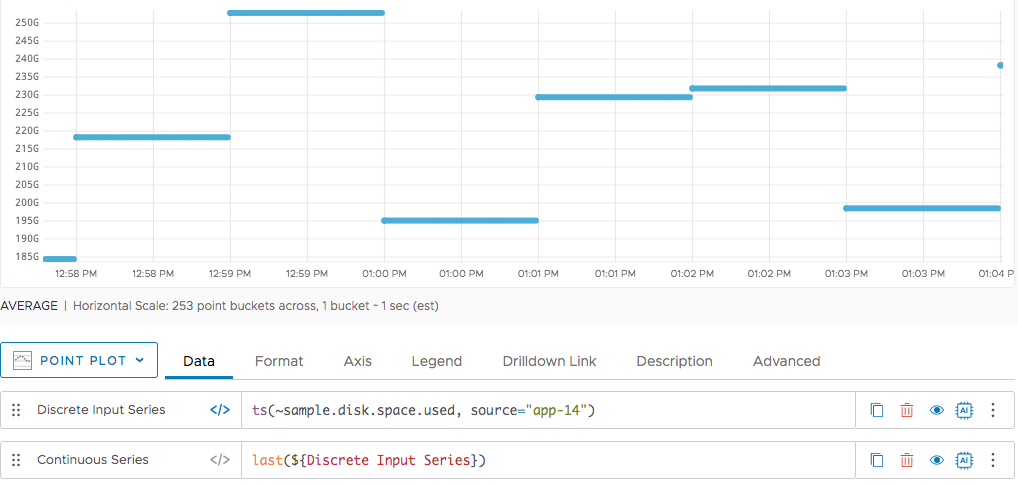Understand discrete and continuous time series and when the query engine performs interpolation.

Tanzu Observability by Wavefront supports both discrete and continuous time series. Understanding how to work with them is important for best performance.

## Basics

Many queries operate on and return data as one or more time series. Each time series is a unique sequence of data points that consists of a data value and a timestamp.

• A discrete time series consists of data points separated by time intervals that are greater than one second. A discrete time series might have:
• A data-reporting interval that is infrequent (e.g., 1 point per minute) or irregular (e.g., whenever a user logs in)
• Gaps where values are missing due to reporting interruptions (e.g., intermittent server or network downtime)
• A continuous time series contains one data point per second. Because we accept and store data at up to 1 second resolution, a continuous time series has a data value corresponding to every moment in time that can be represented on the X-axis of a chart.

## Example

The following chart shows a point plot for the results of two queries. The query labeled Discrete returns multiple time series, each consisting of data points that occur 1 minute apart (at 9:30, 9:31, 9:32, and so on). The query labeled Continuous returns the constant value `160` for every second in the chart.A discrete time series is still discrete when you use a line plot to display it. The following chart shows the same queries, but with the points connected by lines in the display. We’ve used the Color option to show all lines associated with one time series in yellow.## Functions that Preserve Discrete Data

Most query language functions that operate on a discrete time series return a new time series that is also discrete.

Some functions operate on an input time series to produce a new series with the same number of data points at exactly the same times, but with values resulting from some calculation. The result time series will have the same intervals and gaps as the original input time series did. For example:

• The `floor()` function visits each point in a given time series, rounds the point’s data value down to the nearest integer, and assigns the result of that calculation to a new point with a matching timestamp.

Some functions operate on an input time series to produce a new series that has fewer data points. The points in the result series might have different timestamps, different values, or both, and the series typically has wider intervals and gaps. For example:

• The `align()` function groups the input data points into “buckets” and returns a new series that consists of one data point per bucket.
• The `lowpass()` function returns a new series that consists of data points that match just the input points whose values fall below a specified threshold.

## Functions that Create Continuous Data

Certain query language functions and expressions return a new time series that is guaranteed to be continuous (have one data point per second).

Some functions and expressions produce a continuous time series in which a constant value is assigned to every possible data point. For example:

• The expression `160` assigns the value `160` to every data point in a continuous result series.
• The `at()` function obtains a value from a past data point in an input time series and assigns that value to every data point in a continuous result series.

Some functions produce a continuous time series by calculating a value from the timestamp of each data point. For example:

• The `dayOfYear()` function produces a time series by correlating every second of a time line with the day of the year it falls on.

## Functions that Use Interpolation to Create Continuous Data

Certain functions produce a continuous time series by starting with data points from a discrete time series and inserting additional points (1 per second) to fill in the intervals and gaps. You see data every second regardless of the reporting interval of the underlying input data. The process is called interpolation. In the following video, Wavefrount co-founder Clement Pang explains how it works:

For example, the `last()` function produces a new time series that consists of the actual, reported data points from the input series, plus points that are added by interpolation between them. Each interpolated point has the same value as the last reported point before it.

Here’s a point plot showing a discrete series (the red dots) and the points (blue dots) produced by applying `last()`. The points of the discrete series are reported once a minute, and the points between them are all interpolated.Different functions use different techniques to calculate the values of interpolated points. For example:

• When the `last()` function inserts a new point with a particular timestamp, the value assigned to that point is taken from the last actual, reported point before it.
• When the `interpolate()` function inserts a new point with a particular timestamp, the value assigned to that point is an estimate of what the input series would have reported at that time, based on the values of the actual, reported points on either side.

For example:

• Functions such as `last()`, `interpolate()`, and the others summarized below use interpolation to fill in all gaps to produce a result series that is guaranteed to be continuous.
• Standard aggregation functions such as `sum()` and `avg()` use interpolation to fill in specific gaps in an input series before including that series in the aggregation. The result series produced by an aggregation function is normally discrete. Aggregating Time Series gives more details.

## Summary of Functions that Return Continuous Time Series

The following functions always return continuous time series, even when they operate on an input series that is discrete: